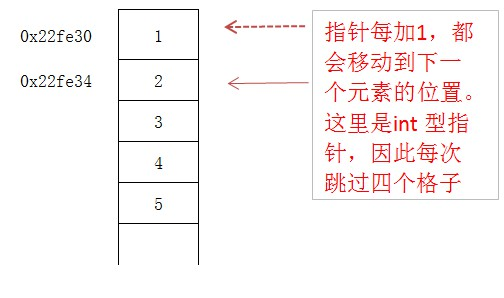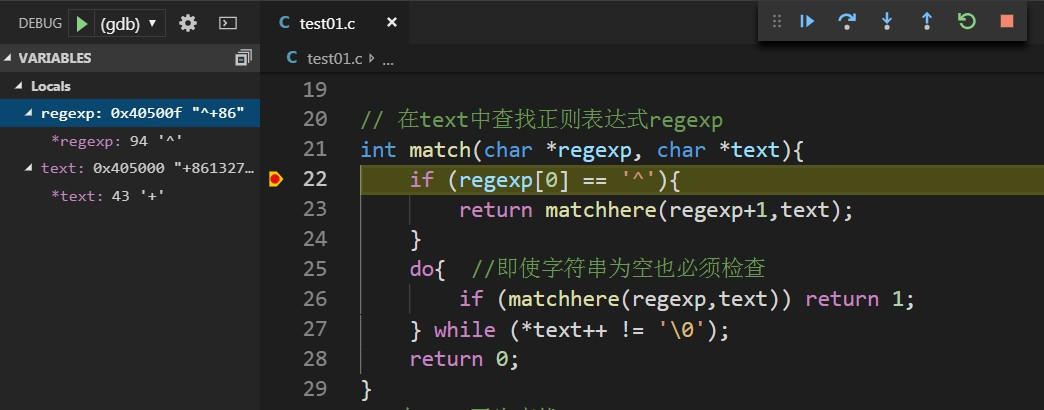# 进阶语法

## 指针与数组

#include <stdio.h>

int main(){
int arr={1,2,3,4,5};

// 依次打印数组每个元素的地址
for (int i = 0; i < 5; i++){
printf("p: %x\n",&arr[i]);
}
return 0;
}


p: 22fe30
p: 22fe34
p: 22fe38
p: 22fe3c
p: 22fe40


### 指针的算术运算

#include <stdio.h>

int main(){
int arr={1,2,3,4,5};

// 声明指针p，指向数组的首元素
int *p = &arr;

// 将指针变量加1，表示偏移一个单位

return 0;
}


arr=1  address=22fe30#include <stdio.h>

int main(){
int arr={1,2,3,4,5};

int *p = &arr;

// 使用指针遍历数组
for (; p <= &arr; p++){
printf("%d\n",*p);
}
return 0;
}


1
2
3
4
5


#include <stdio.h>

int main(){
int arr={1,2,3,4,5};

int *p = &arr;

for (int i = 0; i < 5; i++){
printf("%d\n",p[i]);
}
return 0;
}


### 数组名与指针

#include <stdio.h>

int main(){
int arr={1,2,3,4,5};

int *p = &arr;

printf("p=%x\n",p);
printf("arr=%x\n",arr);

return 0;
}


p=22fe30
arr=22fe30


1. 类型不同。如上，变量p是指针类型，变量arr是数组类型
2. 性质不同。p是变量，可以修改值，重新指向其他地址。arr内部保存的指针是个常量，不能修改和运算。
3. 数组类型可以使用sizeof运算，求得整个数组的内存大小，而对指针p进行sizeof运算，只能得到当前指针所占用的内存大小。

    int arr={1,2,3,4,5};

// 直接使用数组名对指针变量进行初始化，省略&arr的写法，效果是同等的
int *p = arr;


// 形参声明为数组类型：char ch[] ，没用！
// 实际上仍然会退化为指针，编译器不允许在函数传参时，对数组内容进行复制操作，无法实现值传递
// 因此，ch实际上是一个char *类型的指针而已
void convstr(char ch[], int flags);


#include <stdio.h>

void test(int a[]){
// 真正的数组类型，是不能进行指针运算的
// 因此a不是一个数组类型，它就是个指针类型
printf("a=%x\n",a++);
}

int main(){
int arr={1,2,3,4,5};
test(arr);
return 0;
}


## 指针与字符串

#include <stdio.h>

int main(){
// 使用字符串指针表示字符串
char *greet = "hello, Alex";

printf("%s\n",greet);
return 0;
}


address=404000
hello, Alex


#include <stdio.h>

int main(){
char *str1 = "hello, Alex";
char str2[] = "hello, Alice";

str1 = 'f';  //报错，不可修改
str2 = 'f';

printf("%s\n",str1);
printf("%s\n",str2);
return 0;
}


### 字符串的进阶

#include <stdio.h>

int len(char *str){
int i = 0;
for (; *str !='\0'; str++,i++);
return i;
}

int main(){
char *str1 = "hello,Alex";
char str2[] = "hello,Alice";

printf("%d\n",len(str1));
printf("%d\n",len(str2));
return 0;
}


10
11


#### 实现简单正则表达式匹配器

c 匹配任意字母c
. 匹配任意单个字符
^ 匹配字符串的开头
$ 匹配字符串的结尾 * 匹配前一个字符的0个或多个出现 #include <stdio.h> int match(char *regexp, char *text); int matchhere(char *regexp,char *text); int matchstar(int c,char *regexp,char *text); // 创建main函数，测试match函数的功能，其返回1表示匹配成功，0表示无匹配 int main(){ char *str1 = "+8613277880066"; // 检测字符串str1是否以"+86"开头 printf("%d\n",match("^+86",str1)); // 检测字符串str1尾部是否包含"66"子串 printf("%d\n",match("66$",str1));
// 字符串str1中是否包含子串"132"
printf("%d\n",match("132",str1));
// 是否包含3x2样式的子串，x是单个任意字符，这里不包含
printf("%d\n",match("3.2",str1));
return 0;
}

// 在text中查找正则表达式regexp
int match(char *regexp, char *text){
if (regexp == '^'){
return matchhere(regexp+1,text);
}
do{  //即使字符串为空也必须检查
if (matchhere(regexp,text)) return 1;
} while (*text++ != '\0');
return 0;
}
// 在text开头查找regexp
int matchhere(char *regexp,char *text){
if (regexp=='\0') return 1;
if (regexp=='*') {
return matchstar(regexp,regexp+2,text);
}

if (regexp=='\$' && regexp == '\0') {
return *text == '\0';
}

if (*text !='\0' && (regexp == '.' || regexp==*text)) {
return matchhere(regexp+1,text+1);
}
return 0;
}

int matchstar(int c,char *regexp,char *text){
do{   // 通配符* 匹配零个或多个实例
if (matchhere(regexp,text)) return 1;
} while (*text!='\0' && (*text++ == c || c == '.'));
return 0;
}


1
1
1
1
0## 指针常量与常量指针

### 指针常量

    int n = 7;
int l = 10;

//声明并初始化指针常量
int* const p1 = &n;
p1 = &l; // 错误，无法编译！指针常量不能再指向其他地址

// 普通指针，可以指向其他地址
int *p2 = &n;
p2 = &l;


	int n = 7;
int* const p1 = &n;
//可使用解引用符，修改指针常量所指向的内存空间的值
*p1 = 1;	//相当于n=1


	int n = 7;
int *const p1 = &n;


### 常量指针

    int n = 7;
int l = 10;

//声明常量指针
const int *p1 = &n;
*p1 = 0; // 错误，无法编译！不能修改所指向的内容

p1 = &l; //它可以再指向其他地址


### 指向常量的常量指针

    int n = 7;
int l = 10;

//声明指向常量的常量指针
const int* const p1 = &n;
*p1 = 0; // 错误! 不能修改指向的内容
p1 = &l; //错误! 不能重新指向新地址


# 欢迎关注我的公众号：编程之路从0到106-13653

#### 程序员C语言快速上手——基础篇（五）01-173384

#### FileNotFoundError: Could not find module 'xxx.dll'. Try using the full path with constructor syntax.

11-062840

#### 改变隐式加载dll路径的方法(不修改环境变量)

11-07

05-056535

#### 一个年薪一百万的程序员：技术进阶之路

04-10966

#### 在公司的头两年 C/C++程序员成长之路

12-111297

#### C语言程序员必读的5本书©️2020 CSDN 皮肤主题: 技术黑板 设计师: CSDN官方博客点击重新获取扫码支付1.余额是钱包充值的虚拟货币，按照1:1的比例进行支付金额的抵扣。
2.余额无法直接购买下载，可以购买VIP、C币套餐、付费专栏及课程。余额充值